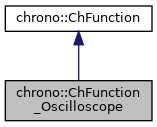chrono::ChFunction_Oscilloscope Class Reference

## Description

Oscilloscope function.

y = interpolation of array of (x,y) data, where (x,y) points must be inserted one after the other, strictly with a fixed dx interval. After a maximum amount of recordable points is reached, the firsts are deleted. Note: differently from ChFunction_Recorder, this function does not allow not-uniform dx spacing between points, but may be faster and simpler to use in many cases.

#include <ChFunction_Oscilloscope.h>

Inheritance diagram for chrono::ChFunction_Oscilloscope:[legend]
Collaboration diagram for chrono::ChFunction_Oscilloscope:[legend]

## Public Member Functions

ChFunction_Oscilloscope (const ChFunction_Oscilloscope &other)

virtual ChFunction_OscilloscopeClone () const override
"Virtual" copy constructor (covariant return type).

virtual FunctionType Get_Type () const override
Return the unique function type identifier.

virtual double Get_y (double x) const override
Return the y value of the function, at position x.

void AddLastPoint (double mx, double my)
Add a point at the head (right side of point array). More...

void Reset ()
Reset the array or recorded points.

std::list< double > & GetPointList ()
Access directly the list of points.

double Get_dx () const
Get the dx spacing between recorded points. It is assumed uniform!

void Set_dx (double mdx)
Set the dx spacing between recorded points. It is assumed uniform!

int Get_max_amount () const
Get the maximum amount of points which can be entered (after this, the first one will be deleted, as in a FIFO)

void Set_max_amount (int mnum)
Set the maximum amount of points which can be entered (after this, the first one will be deleted, as in a FIFO)

double Get_amount () const
Get the amount of recorded points.

virtual void Estimate_x_range (double &xmin, double &xmax) const override
Return an estimate of the range of the function argument. More...

virtual void ArchiveOUT (ChArchiveOut &marchive) override
Method to allow serialization of transient data to archives.

virtual void ArchiveIN (ChArchiveIn &marchive) override
Method to allow de-serialization of transient data from archives.Public Member Functions inherited from chrono::ChFunction
ChFunction (const ChFunction &other)

virtual double Get_y_dx (double x) const
Return the dy/dx derivative of the function, at position x. More...

virtual double Get_y_dxdx (double x) const
Return the ddy/dxdx double derivative of the function, at position x. More...

virtual double Get_weight (double x) const
Return the weight of the function (useful for applications where you need to mix different weighted ChFunctions)

virtual void Estimate_y_range (double xmin, double xmax, double &ymin, double &ymax, int derivate) const
Return an estimate of the range of the function value. More...

virtual double Get_y_dN (double x, int derivate) const
Return the function derivative of specified order at the given point. More...

virtual void Update (const double x)
Update could be implemented by children classes, ex. to launch callbacks.

virtual double Compute_max (double xmin, double xmax, double sampling_step, int derivate) const
Compute the maximum of y(x) in a range xmin-xmax, using a sampling method.

virtual double Compute_min (double xmin, double xmax, double sampling_step, int derivate) const
Compute the minimum of y(x) in a range xmin-xmax, using a sampling method.

virtual double Compute_mean (double xmin, double xmax, double sampling_step, int derivate) const
Compute the mean value of y(x) in a range xmin-xmax, using a sampling method.

virtual double Compute_sqrmean (double xmin, double xmax, double sampling_step, int derivate) const
Compute the square mean val. of y(x) in a range xmin-xmax, using sampling.

virtual double Compute_int (double xmin, double xmax, double sampling_step, int derivate) const
Compute the integral of y(x) in a range xmin-xmax, using a sampling method.

virtual double Get_Ca_pos () const
Computes the positive acceleration coefficient (inherited classes should customize this).

virtual double Get_Ca_neg () const
Compute the positive acceleration coefficient (inherited classes should customize this).

virtual double Get_Cv () const
Compute the speed coefficient (inherited classes must customize this).

virtual int HandleNumber () const
Return the number of handles of the function.

virtual bool HandleAccess (int handle_id, double mx, double my, bool set_mode)
Get the x and y position of handle, given identifier. More...

virtual int FilePostscriptPlot (ChFile_ps *m_file, int plotY, int plotDY, int plotDDY)
Plot function in graph space of the ChFile_ps postscript file where zoom factor, centering, color, thickness etc. More...

virtual int FileAsciiPairsSave (ChStreamOutAscii &m_file, double xmin=0, double xmax=1, int msamples=200)
Save function as X-Y pairs separated by space, with CR at each pair, into an ASCII file. More...Public Types inherited from chrono::ChFunction
enum  FunctionType {
FUNCT_CUSTOM, FUNCT_CONST, FUNCT_CONSTACC, FUNCT_DERIVE,
FUNCT_FILLET3, FUNCT_INTEGRATE, FUNCT_MATLAB, FUNCT_MIRROR,
FUNCT_MOCAP, FUNCT_NOISE, FUNCT_OPERATION, FUNCT_OSCILLOSCOPE,
FUNCT_POLY, FUNCT_POLY345, FUNCT_RAMP, FUNCT_RECORDER,
FUNCT_REPEAT, FUNCT_SEQUENCE, FUNCT_SIGMA, FUNCT_SINE,
FUNCT_LAMBDA
}
Enumeration of function types.

## Member Function Documentation

 void chrono::ChFunction_Oscilloscope::AddLastPoint ( double mx, double my )

Note that it is user's responsibility to add points which are spaced uniformly (by dx) on the X axis! No checks are done on the correctness of the dx spacing, except that if you enter a point whose mx is less than the mx of the one you previously entered, the array is cleared.

## ◆ Estimate_x_range()

 void chrono::ChFunction_Oscilloscope::Estimate_x_range ( double & xmin, double & xmax ) const
overridevirtual

Return an estimate of the range of the function argument.

(Can be used for automatic zooming in a GUI)

Reimplemented from chrono::ChFunction.

The documentation for this class was generated from the following files:
• /builds/uwsbel/chrono/src/chrono/motion_functions/ChFunction_Oscilloscope.h
• /builds/uwsbel/chrono/src/chrono/motion_functions/ChFunction_Oscilloscope.cpp